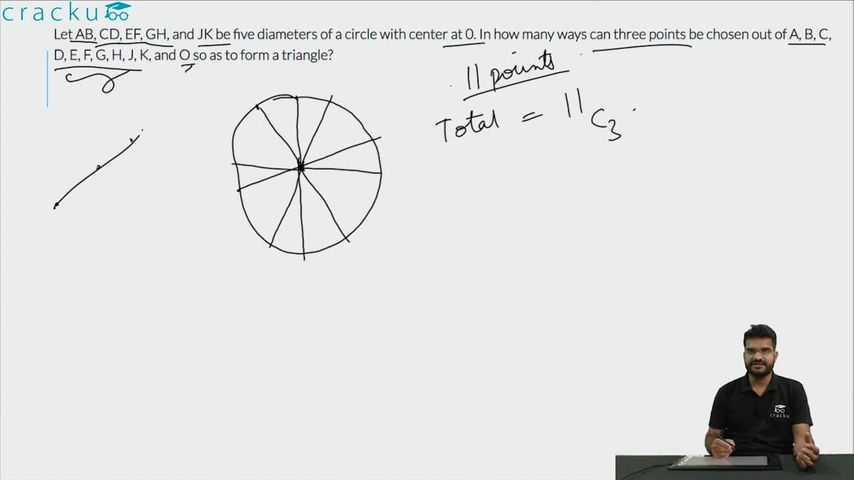Question 95

# Let AB, CD, EF, GH, and JK be five diameters of a circle with center at 0. In how many ways can three points be chosen out of A, B, C, D, E, F, G, H, J, K, and O so as to form a triangle?

Solution

The total number of given points are 11. (10 on circumference and 1 is the center)
So total possible triangles = 11C3 = 165.
However, AOB, COD, EOF, GOH, JOK lie on a straight line. Hence, these 5 triangles are not possible. Thus, the required number of triangles = 165 - 5 = 160

### View Video Solution